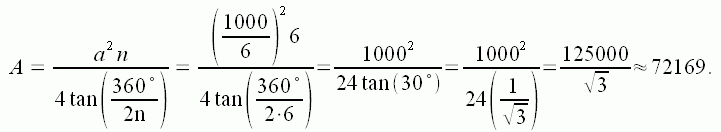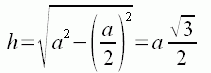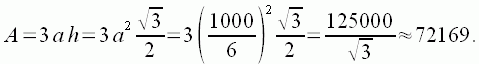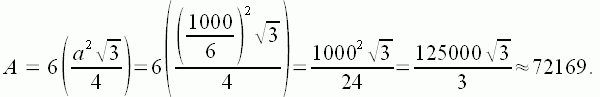SEARCH HOMEMath Central Quandaries & QueriesQuestion from josh, a student: I have to work out the area of a hexagon when I know that the perimeter of it is 1000m. I have tried many different ways but I get different answers, like with the formula I get 72,000 but when I do the split it into triangles thingy I get 59,000 odd.PLEASE tell me which is the right answer!!Hi Josh.

I get a bit more than 72 000 no matter how I solve the problem.

1. Using the formula for a regular polygon.
In the formula I wrote about for any regular polygon, we would first say that the side length a = 1000/6, and the number of sides n = 6, so:2. Using a geometric transformation of the hexagon into a rectangle.
Geometrically, the equivalent rectangle is 3a units wide and h units high. But h is found using Pythagorus, so:thus3. Breaking the polygon into six equilateral triangles.
If you recognize that a hexagon is made of 6 congruent equilateral triangles, then you can use the formula for the area of an equilateral triangle and multiply by 6:So all three ways I approached the problem, I got the same result.

Hope this helps,
Stephen La Rocque.Math Central is supported by the University of Regina and The Pacific Institute for the Mathematical Sciences.